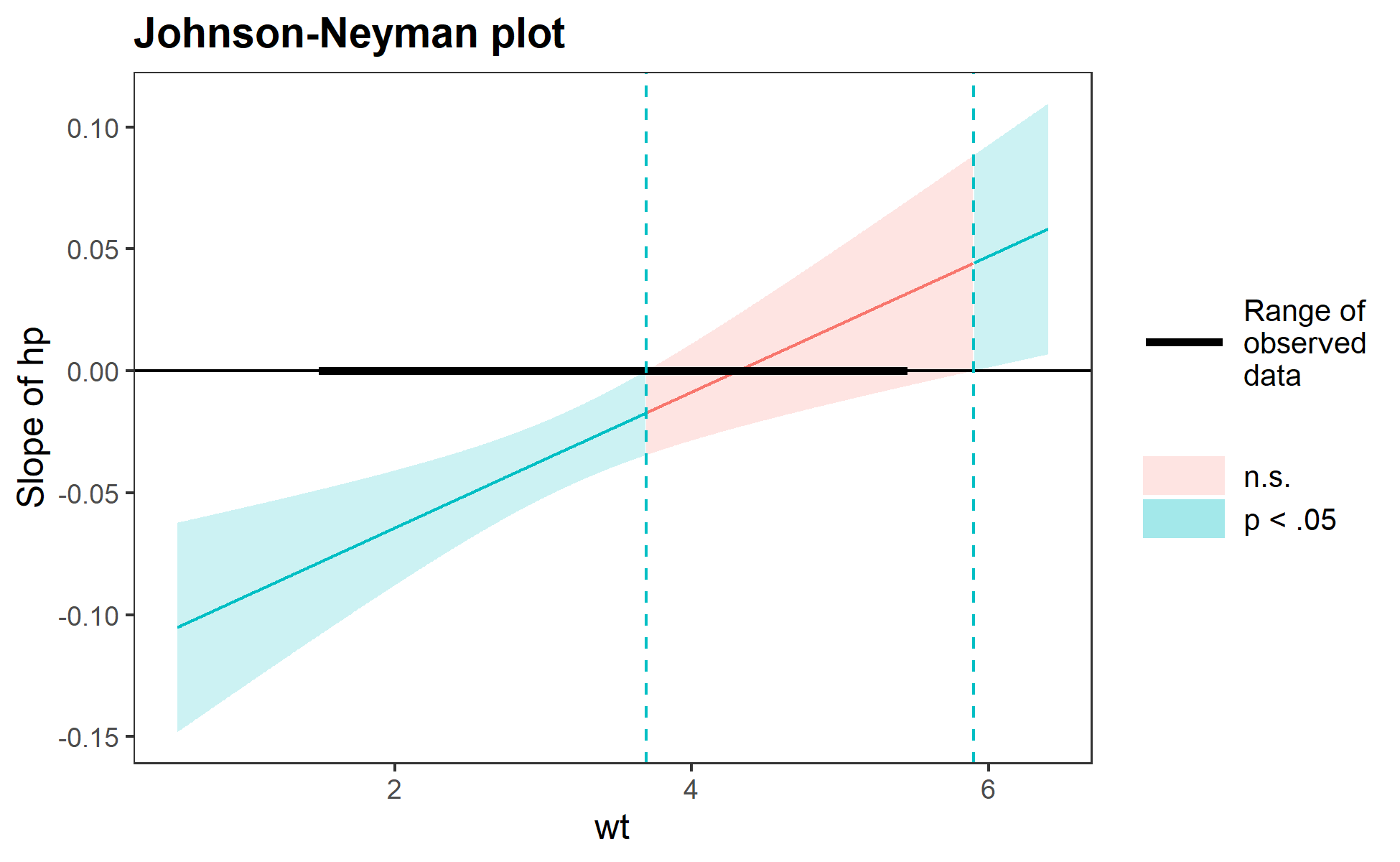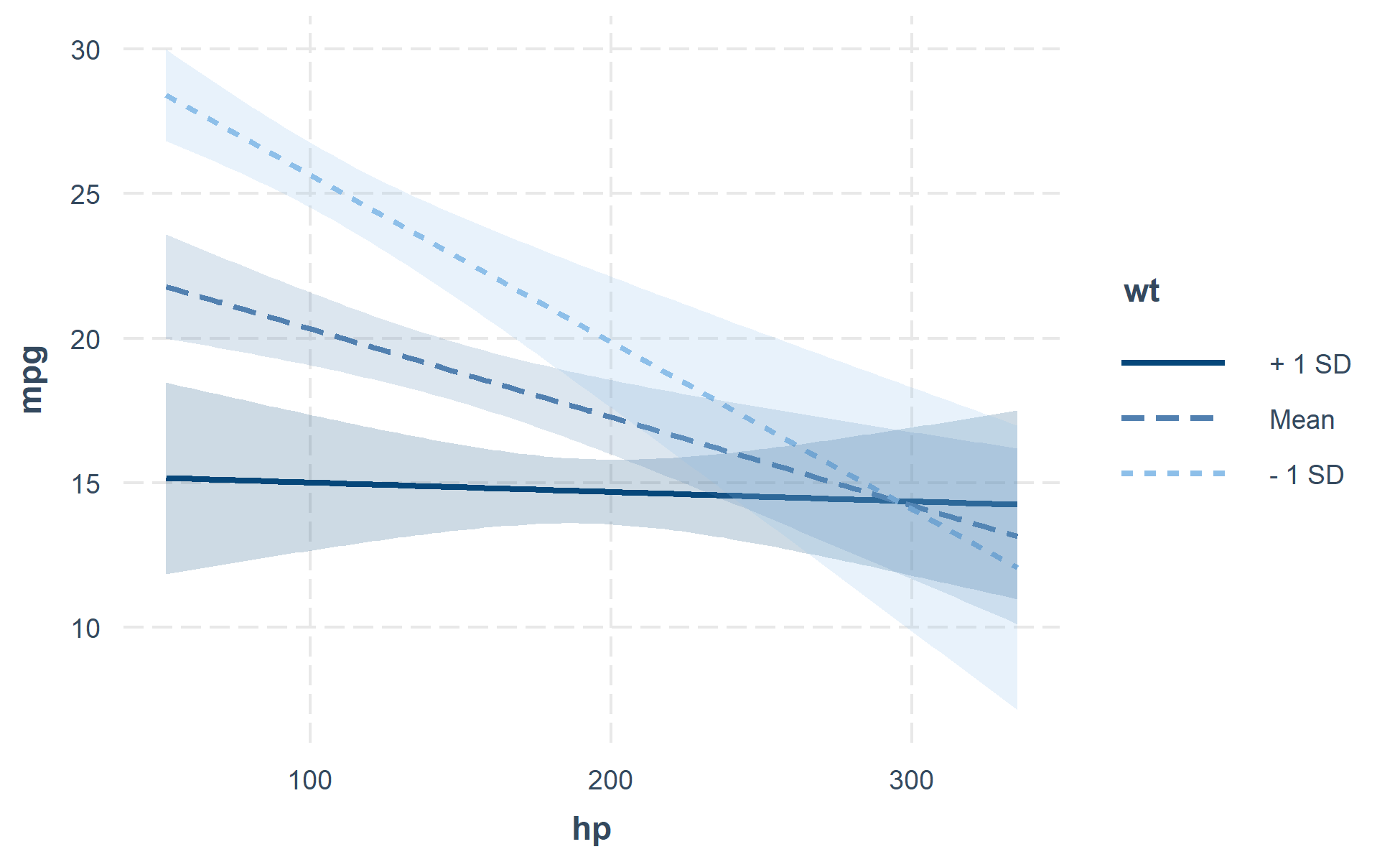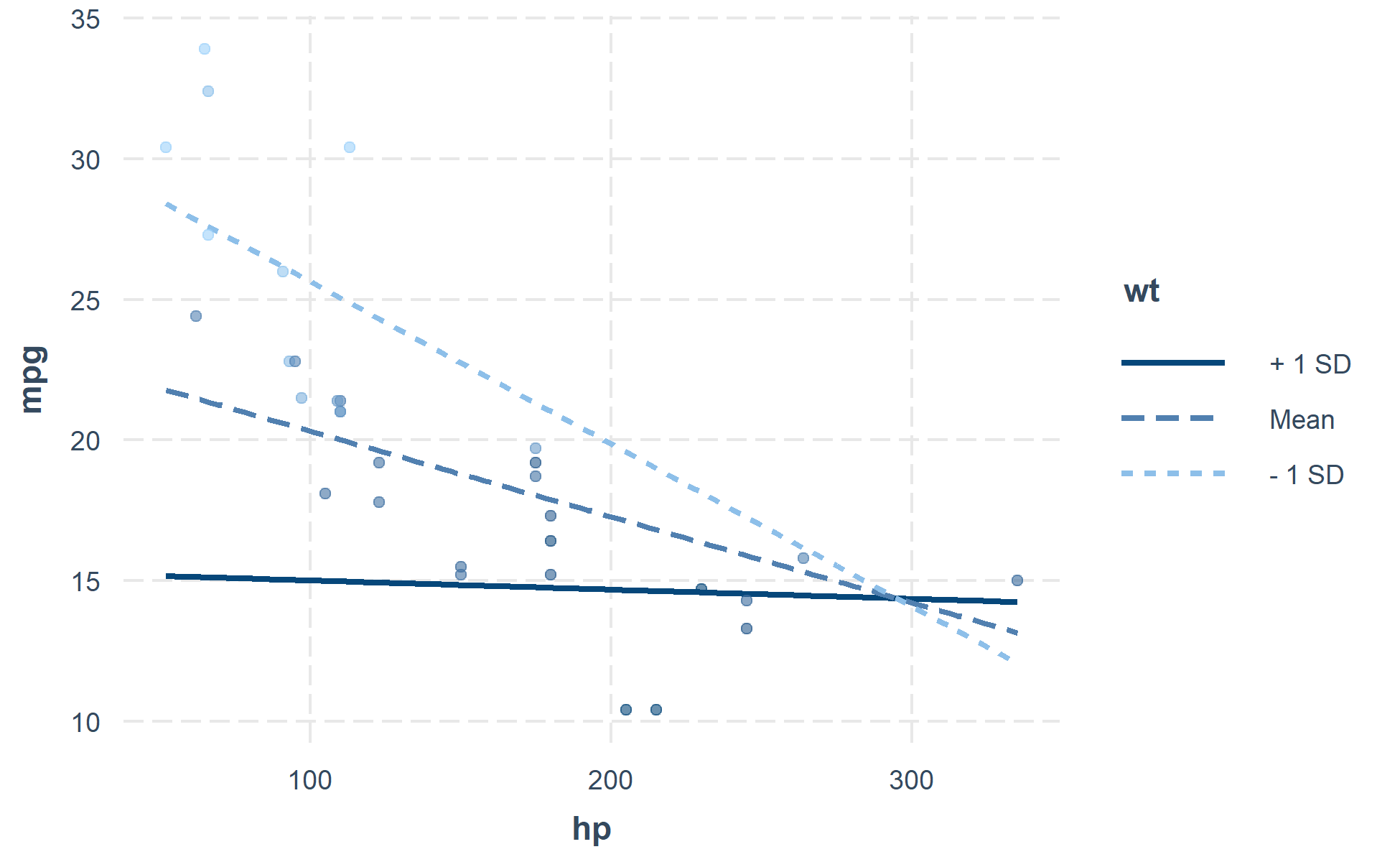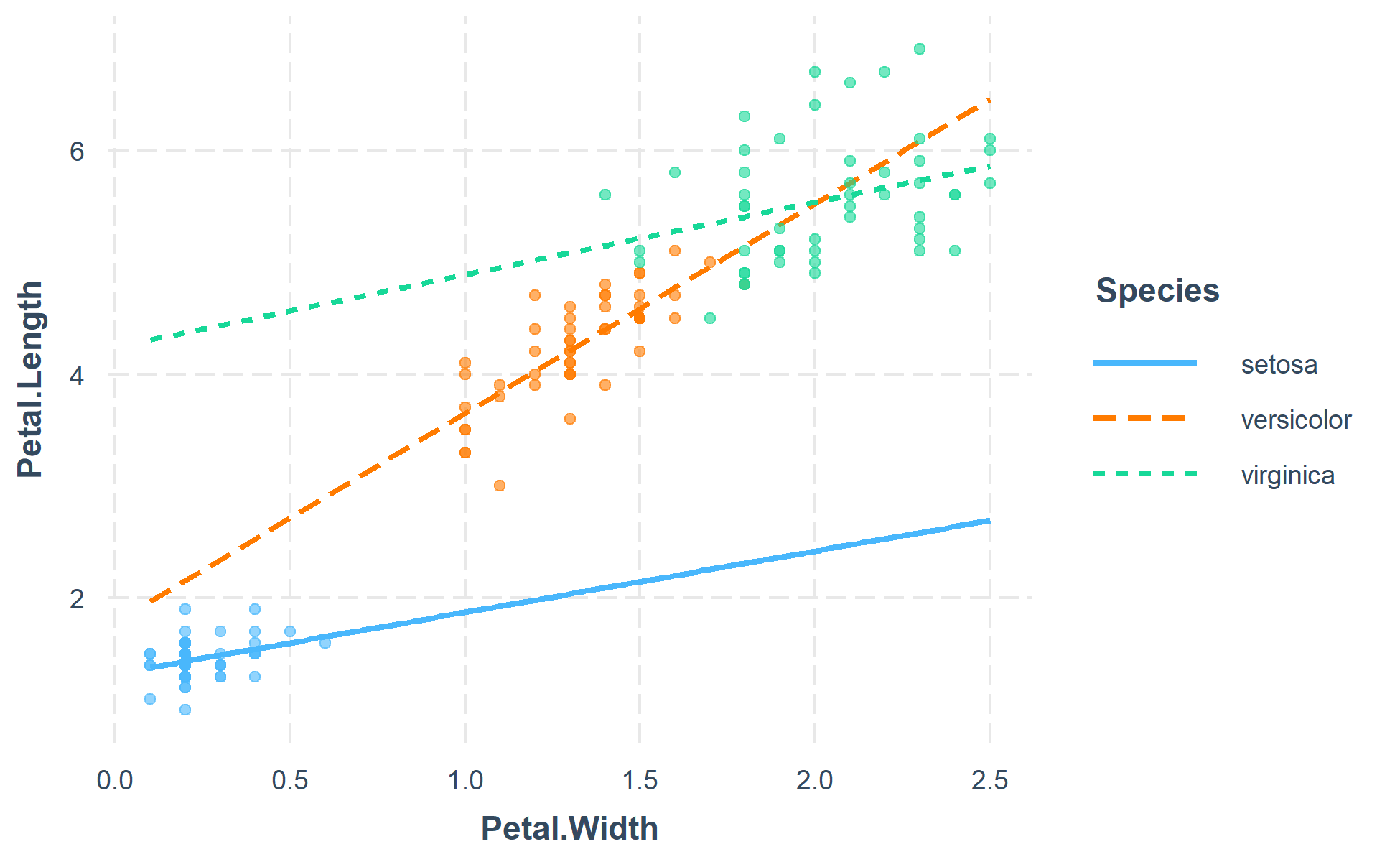# Comprehensive, User-Friendly Toolkit for Probing Interactions

A suite of functions for conducting and interpreting analysis of statistical interaction in regression models that was formerly part of the 'jtools' package. Functionality includes visualization of two- and three-way interactions among continuous and/or categorical variables as well as calculation of "simple slopes" and Johnson-Neyman intervals (see e.g., Bauer & Curran, 2005 ). These capabilities are implemented for generalized linear models in addition to the standard linear regression context.

This package consists of a number of tools that pertain to the analysis and exploration of statistical interactions in the context of regression. Some of these features, especially those that pertain to visualization, are not exactly impossible to do oneself but are tedious and error-prone when done “by hand.” Most things in `interactions` were once part of the `jtools` package and have been spun off to this package for clarity and simplicity.

Quick rundown of features:

• simple slopes analysis
• calculation of Johnson-Neyman intervals
• visualization of predicted and observed values using `ggplot2`

All of these are implemented in a consistent interface designed to be as simple as possible with tweaks and guts available to advanced users. GLMs, models from the `survey` package, and multilevel models from `lme4` are fully supported as is visualization for Bayesian models from `rstanaram` and `brms`.

## Installation

For the moment, the package has just been submitted to CRAN and may not yet be available as you read this. If that is the case, please install from Github.

## Usage

Unless you have a really keen eye and good familiarity with both the underlying mathematics and the scale of your variables, it can be very difficult to look at the output of regression model that includes an interaction and actually understand what the model is telling you.

This package contains several means of aiding understanding and doing statistical inference with interactions.

### Johnson-Neyman intervals and simple slopes analysis

The “classic” way of probing an interaction effect is to calculate the slope of the focal predictor at different values of the moderator. When the moderator is binary, this is especially informative—e.g., what is the slope for men vs. women? But you can also arbitrarily choose points for continuous moderators.

With that said, the more statistically rigorous way to explore these effects is to find the Johnson-Neyman interval, which tells you the range of values of the moderator in which the slope of the predictor is significant vs.  nonsignificant at a specified alpha level.

The `sim_slopes` function will by default find the Johnson-Neyman interval and tell you the predictor’s slope at specified values of the moderator; by default either both values of binary predictors or the mean and the mean +/- one standard deviation for continuous moderators.

``````#> JOHNSON-NEYMAN INTERVAL
#>
#> When wt is OUTSIDE the interval [3.69, 5.90], the slope of hp is p <
#> .05.
#>
#> Note: The range of observed values of wt is [1.51, 5.42]
````````````#> SIMPLE SLOPES ANALYSIS
#>
#> Slope of hp when wt = 4.20 (+ 1 SD):
#>
#>   Est.   S.E.   t val.      p
#> ------  -----  -------  -----
#>  -0.00   0.01    -0.31   0.76
#>
#> Slope of hp when wt = 3.22 (Mean):
#>
#>   Est.   S.E.   t val.      p
#> ------  -----  -------  -----
#>  -0.03   0.01    -4.07   0.00
#>
#> Slope of hp when wt = 2.24 (- 1 SD):
#>
#>   Est.   S.E.   t val.      p
#> ------  -----  -------  -----
#>  -0.06   0.01    -5.66   0.00
``````

The Johnson-Neyman plot can really help you get a handle on what the interval is telling you, too. Note that you can look at the Johnson-Neyman interval directly with the `johnson_neyman` function.

The above all generalize to three-way interactions, too.

### Visualizing interaction effects

This function plots two- and three-way interactions using `ggplot2` with a similar interface to the aforementioned `sim_slopes` function. Users can customize the appearance with familiar `ggplot2` commands. It supports several customizations, like confidence intervals.You can also plot the observed data for comparison:The function also supports categorical moderators—plotting observed data in these cases can reveal striking patterns.You may also combine the plotting and simple slopes functions by using `probe_interaction`, which calls both functions simultaneously. Categorical by categorical interactions can be investigated using the `cat_plot` function.

## Contributing

I’m happy to receive bug reports, suggestions, questions, and (most of all) contributions to fix problems and add features. I prefer you use the Github issues system over trying to reach out to me in other ways. Pull requests for contributions are encouraged.

Please note that this project is released with a Contributor Code of Conduct. By participating in this project you agree to abide by its terms.

# interactions 1.1.0

## New function: `sim_margins()`

This is, as the name suggests, related to `sim_slopes()`. However, instead of slopes, what is being estimated are marginal effects. In the case of OLS linear regression, this is basically the same thing. The slope in OLS is the expected change in the outcome for each 1-unit increase in the predictor. For other models, however, the actual change in the outcome when there's a 1-unit increase in a variable depends on the level of other covariates and the initial value of the predictor. In a logit model, for instance, the change in probability will be different if the initial probability was 50% (could go quite a bit up or down) than if it was 99.9% (can't go up).

`sim_margins()` uses the `margins` package under the hood to estimate marginal effects. Unlike `sim_slopes()`, in which by default all covariates not involved in the interaction are mean-centered, in `sim_margins()` these covariates are always left at their observed values because they influence the level of the marginal effect. Instead, the marginal effect is calculated with the covariates and focal predictor (`pred`) at their observed values and the moderator(s) held at the specified values (e.g., the mean and 1 standard deviation above/below the mean). I advise using `sim_margins()` rather than `sim_slopes()` when analyzing models other than OLS regression.

## Bug fixes

• `interact_plot()` and `cat_plot()` now respect the user's selection of `outcome.scale`; in 1.0.0, it always plotted on the response scale. (#12)
• The `modx.values` argument is now better documented to explain that you may use it to specify the exact values you want. Thanks to Jakub Lysek for asking the question that prompted this. (#8)
• `modx.values` now accepts `"mean-plus-minus"` as a manual specification of the default auto-calculated values for continuous moderators. `NULL` still defaults to this, but you can now make this explicit in your code if desired for clarity or to guard against future changes in the default behavior.
• Users are now warned when `modx.values` or `mod2.values` include values outside the observed range of the `modx`/`mod2`. (#9)
• Users are now warned when `pred`, `modx`, and `mod2` are not all involved in an interaction with each other in the provided model. (#10)
• `cat_plot()` was ignoring `mod2.values` arguments but now works properly. (#17)
• Missing values in the original data are now handled better in `interact_plot()` and `cat_plot()`.
• `sim_slopes()` now handles non-syntactic variable names better.
• `interactions` now requires you to have a relatively new version of `rlang`. Users with older versions were experiencing cryptic errors. (#15)

• `interact_plot()` and `cat_plot()` now have an `at` argument for more granular control over the values of covariates.
• `sim_slopes()` now allows for custom specification of robust standard error estimators via providing a function to `v.cov` and arguments to `v.cov.args`.

# interactions 1.0.0

This is the first release, but a look at the NEWS for `jtools` prior to its version 2.0.0 will give you an idea of the history of the functions in this package.

What follows is an accounting of changes to functions in this package since they were last in `jtools`.

• Plots made by `interactions` now have a new theme, which you can use yourself, called `theme_nice()` (from the `jtools` package). The previous default, `theme_apa()`, is still available but I don't like it as a default since I don't think the APA has defined the nicest-looking design guidelines for general use.
• `interact_plot()` now has appropriate coloring for observed data when the moderator is numeric (#1). In previous versions I had to use a workaround that involved tweaking the alpha of the observed data points.
• `interact_plot()` and `cat_plot()` now use tidy evaluation for the `pred`, `modx`, and `mod2` arguments. This means you can pass a variable that contains the name of `pred`/`modx`/`mod2`, which is most useful if you are creating a function, for loop, etc. If using a variable, put a `!!` from the `rlang` package before it (e.g., `pred = !! variable`). For most users, these changes will not affect their usage.
• `sim_slopes()` no longer prints coefficient tables as data frames because this caused RStudio notebook users issues with the output not being printed to the console and having the notebook format them in less-than-ideal ways. The tables now have a markdown format that might remind you of Stata's coefficient tables. Thanks to Kim Henry for contacting me about this.

## Use partial residuals for plotting

One negative when visualizing predictions alongside original data with `interact_plot()` or similar tools is that the observed data may be too spread out to pick up on any patterns. However, sometimes your model is controlling for the causes of this scattering, especially with multilevel models that have random intercepts. Partial residuals include the effects of all the controlled-for variables and let you see how well your model performs with all of those things accounted for.

You can plot partial residuals instead of the observed data in `interact_plot()` and `cat_plot()` via the argument `partial.residuals = TRUE`.

## Important changes to `make_predictions()` and removal of `plot_predictions()`

In the `jtools` 1.0.0 release, I introduced `make_predictions()` as a lower-level way to emulate the functionality of `effect_plot()`, `interact_plot()`, and `cat_plot()`. This would return a list object with predicted data, the original data, and a bunch of attributes containing information about how to plot it. One could then take this object, with class `predictions`, and use it as the main argument to `plot_predictions()`, which was another new function that creates the plots you would see in `effect_plot()` et al.

I have simplified `make_predictions()` to be less specific to those plotting functions and eliminated `plot_predictions()`, which was ultimately too complex to maintain and caused problems for separating the interaction tools into a separate package. `make_predictions()` by default simply creates a new data frame of predicted values along a `pred` variable. It no longer accepts `modx` or `mod2` arguments. Instead, it accepts an argument called `at` where a user can specify any number of variables and values to generate predictions at. This syntax is designed to be similar to the `predictions`/`margins` packages. See the `jtools` documentation for more info on this revised syntax.

# Reference manual

install.packages("interactions")

1.1.5 by Jacob A. Long, 4 months ago

https://interactions.jacob-long.com

Report a bug at https://github.com/jacob-long/interactions/issues

Browse source code at https://github.com/cran/interactions

Authors: Jacob A. Long [aut, cre]

Documentation:   PDF Manual